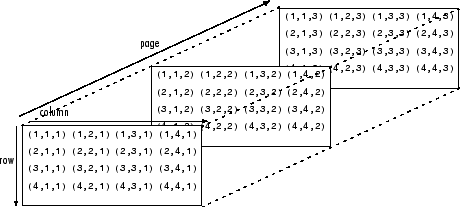# pagectranspose

Page-wise complex conjugate transpose

## Syntax

``Y = pagectranspose(X)``

## Description

example

````Y = pagectranspose(X)` applies the complex conjugate transpose to each page of N-D array `X`. Each page of the output `Y(:,:,i)` is the conjugate transpose of the corresponding page in `X`, as in `X(:,:,i)'`.```

## Examples

collapse all

Create a 3-D complex array `A`, and then use `pagectranspose` to find the complex conjugate transpose of each page of the array.

```r = repelem(1:3,3,1); A = cat(3,r,2*r,3*r); A = A + 1i```
```A = A(:,:,1) = 1.0000 + 1.0000i 2.0000 + 1.0000i 3.0000 + 1.0000i 1.0000 + 1.0000i 2.0000 + 1.0000i 3.0000 + 1.0000i 1.0000 + 1.0000i 2.0000 + 1.0000i 3.0000 + 1.0000i A(:,:,2) = 2.0000 + 1.0000i 4.0000 + 1.0000i 6.0000 + 1.0000i 2.0000 + 1.0000i 4.0000 + 1.0000i 6.0000 + 1.0000i 2.0000 + 1.0000i 4.0000 + 1.0000i 6.0000 + 1.0000i A(:,:,3) = 3.0000 + 1.0000i 6.0000 + 1.0000i 9.0000 + 1.0000i 3.0000 + 1.0000i 6.0000 + 1.0000i 9.0000 + 1.0000i 3.0000 + 1.0000i 6.0000 + 1.0000i 9.0000 + 1.0000i ```
`B = pagectranspose(A)`
```B = B(:,:,1) = 1.0000 - 1.0000i 1.0000 - 1.0000i 1.0000 - 1.0000i 2.0000 - 1.0000i 2.0000 - 1.0000i 2.0000 - 1.0000i 3.0000 - 1.0000i 3.0000 - 1.0000i 3.0000 - 1.0000i B(:,:,2) = 2.0000 - 1.0000i 2.0000 - 1.0000i 2.0000 - 1.0000i 4.0000 - 1.0000i 4.0000 - 1.0000i 4.0000 - 1.0000i 6.0000 - 1.0000i 6.0000 - 1.0000i 6.0000 - 1.0000i B(:,:,3) = 3.0000 - 1.0000i 3.0000 - 1.0000i 3.0000 - 1.0000i 6.0000 - 1.0000i 6.0000 - 1.0000i 6.0000 - 1.0000i 9.0000 - 1.0000i 9.0000 - 1.0000i 9.0000 - 1.0000i ```

## Input Arguments

collapse all

Input array, specified as a multidimensional array.

Data Types: `single` | `double` | `int8` | `int16` | `int32` | `int64` | `uint8` | `uint16` | `uint32` | `uint64` | `logical` | `char` | `string` | `struct` | `cell` | `categorical` | `datetime` | `duration` | `calendarDuration`
Complex Number Support: Yes

collapse all

### Array Pages

Page-wise functions like `pagectranspose` operate on 2-D matrices that have been arranged into a multidimensional array. For example, with a 3-D array the elements in the third dimension of the array are commonly called pages since they stack on top of each other like pages in a book. Each page is a matrix that gets operated on by the function.You can also assemble a collection of 2-D matrices into a higher dimensional array, like a 4-D or 5-D array, and in these cases `pagectranspose` still treats the fundamental unit of the array as a 2-D matrix that gets operated on, such as `X(:,:,i,j,k,l)`.

The `cat` function is useful to assemble a collection of matrices into a multidimensional array, and the `zeros` function is useful to preallocate a multidimensional array.

## Tips

• The page-wise complex conjugate transpose is equivalent to permuting the first two dimensions of the array with `permute(conj(X),[2 1 3:ndims(X)])`.